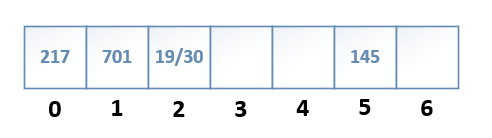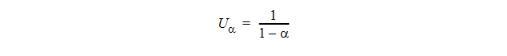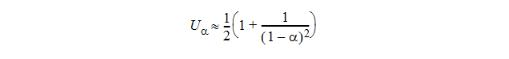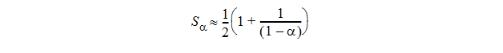#0
0
01. 云栖社区>
2. vivo互联网技术>
3. 博客>
4. 正文

## 你还应该知道的哈希冲突解决策略

2020Labs 2020-05-06 10:36:23 浏览255

## 一、哈希表概述

• md5 : 2^128个值（找一个冲突键，需要哈希大约2 ^ 64个值）

• sha-1：2^160个值（找一个冲突键，需要大约2^80个值）

## 二、哈希冲突

H(K) = 217 % 7 = 0

H(K) = 701 % 7 = 1

H(K) = 19 % 7 = 2

H(K) = 30 % 7 = 2

H(K) = 145 % 7 = 5## 三、冲突解决策略

• 线性探测(Linear probing)

• 双重哈希(Double hashing)

• 随机散列(Random hashing)

• 分离链接(Separate chaining)

### 1、线性探测(Linear probing)

1. 设置 indx = H（K）

2. 如果表位置indx已经包含密钥，则无需插入它。Over

3. 否则，如果表位置indx为空，则在其中插入键。Over

4. 其他碰撞。设置 indx =（indx + 1）mod M.

5. 如果 indx == H（K），则表已满！就只能做哈希表的扩容了

H(K) = 701 % 7 = 1

H(K) = 145 % 7 = 5

H(K) = 217 % 7 = 0

H(K) = 19 % 7 = 2

H(K) = 13 % 7 = 1(冲突) --> 2(已经有值) --> 3(插入位置3)

H(K) = 749 % 7 = 2(冲突) --> 3(已经有值) --> 4(插入位置4)

1. 设置 indx = H（K）

2. 如果表位置indx包含键，则返回FOUND。

4. 否则设置 indx =（indx + 1）modM。

### 2、双重哈希(Double hashing)

1. 设置 indx = H（K）; offset = H 2（K）

2. 如果表位置indx已经包含密钥，则无需插入它。Over

3. 否则，如果表位置 indx 为空，则在其中插入键。Over

4. 其他碰撞。设置 indx =（indx + offset）mod M.

5. 如果 indx == H（K），则表已满！就只能做哈希表的扩容了

### 3、随机散列(Random hashing)

1. 创建以 K 为种子的 RNG。设置indx = RNG.next() mod M。

2. 如果表位置 indx 已经包含密钥，则无需插入它。Over

3. 否则，如果表位置 indx 为空，则在其中插入键。Over

4. 其他碰撞。设置 indx = RNG.next() mod M.

5. 如果已探测所有M个位置，则放弃。就只能做哈希表的扩容了。

### 4、分离链接(Separate chaining)

1. 设置 indx = H（K）

2. 将关键字插入到以 indx 为标题的链接列表中。（首先搜索列表，以避免重复。）

1. 设置 indx = H（K）

2. 使用线性搜索在以 indx 为标题的链表中搜索关键字。

1. 设置 indx = H（K）

2. 删除链接列表中以 indx 为标题的键

• 如果查找/插入失败，则必须穷举搜索表中的链表之一，并且表中链表的平均长度为α。因此，使用单独链接进行插入或不成功查找的比较平均次数为


![](https://oscimg.oschina.net/oscnet/up-d1abf33898b7083dff803fcf6c509158bcf.JPEG)


• 成功查找后，将搜索包含目标密钥的链接列表。除目标密钥外，该列表中平均还有（N-1）/ M个密钥；在找到目标之前，将平均搜索其中一半。因此，使用单独链接成功找到的比较平均次数为


![](https://oscimg.oschina.net/oscnet/up-f2b7ba6592698925f4b91f9bb40b39a6819.JPEG)



## 四、开散列方法 VS 闭散列方法2020Labs
+ 关注